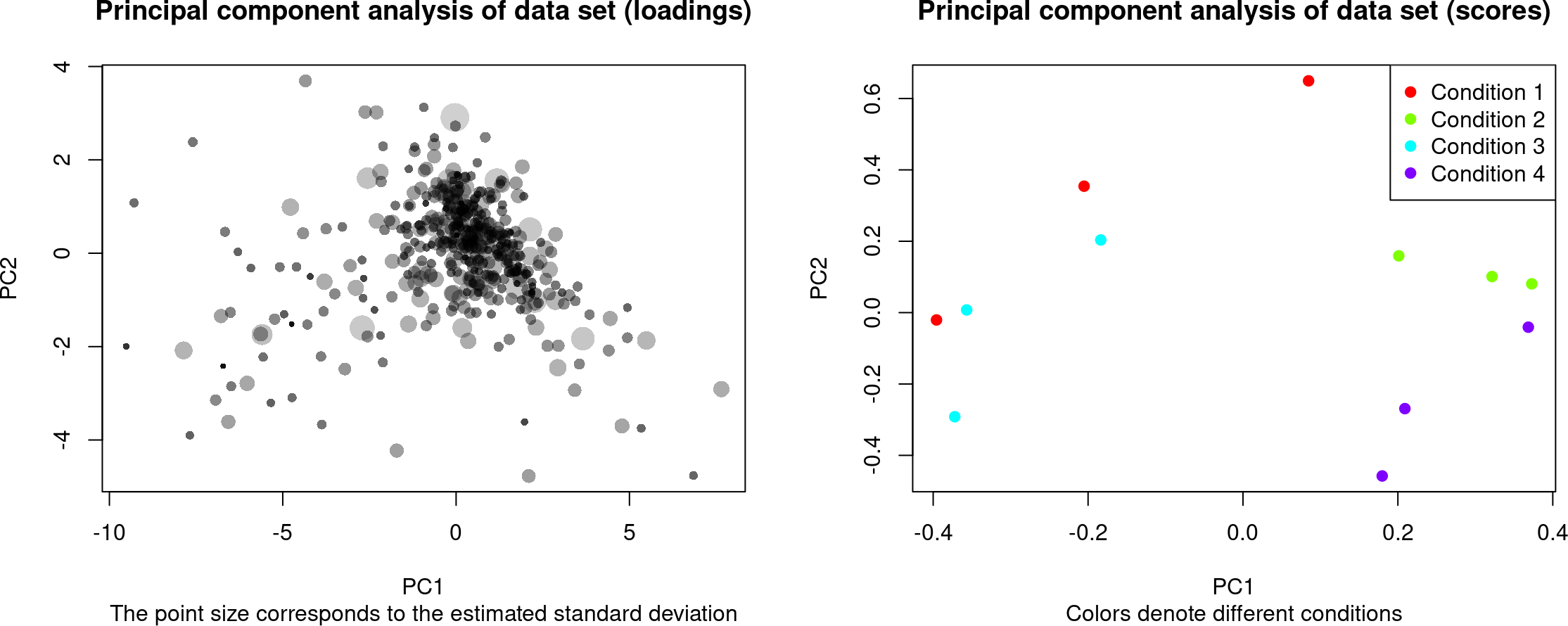# 1 Introduction

Clustering is a method to identify common pattern in highly dimensional data. This can be for example genes or proteins with similar quantitative changes, thus providing insights into the affected biological pathways.

Despite of numerous clustering algorithms, they do not account for feature variance, i.e. the uncertainty in the measurements across the different experimental conditions. VSClust determines the characteristic patterns in high-dimensional data while accounting for feature variance that is given through replicated measurements.

Here, we present an example script to run the full clustering analysis using the `vsclust` library. The same can be done by running the Shiny app (e.g. via its docker image or on ), or the corresponding command line script. For the source code, see .

# 2 Installation and additional packages

Use the common Bioconductor commands for installation:

``````if (!require("BiocManager", quietly = TRUE))
install.packages("BiocManager")
BiocManager::install("vsclust")``````

The full functionality of this vignette can be obtained by additionally installing and loading the packages `matrixStats` and `clusterProfiler`

# 3 Initialization

Here, we define the different parameters for the example data set `protein_expressions`. In the command-line version of VSClust (“runVSClust.R”), they can be given via yaml file.

A. Data sets with different numbers of replicates per condition need to be adapted to contain the same number of columns per condition. These can be done by either removing excess replicates or adding empty columns.

B. We assume the input data to be of the following format: A1, B1, C1, …, A2, B2, C2, …, where letters denote sample type and numbers are the different replicates.

C. If you prefer to estimate feature variance different, use averages and add an estimate for the standard deviation as last column. You will need to set the last option of `PreparedForVSClust` to `FALSE`.

D. If you don’t have replicates, use the same format as in C. and set the standard deviations to 1.

``````#### Input parameters, only read when now parameter file was provided
## All principal parameters for running VSClust can be defined as in the
## shinyapp at computproteomics.bmb.sdu.dk/Apps/VSClust
# name of study
Experiment <- "ProtExample"
# Number of replicates/sample per different experimental condition (sample
# type)
NumReps <- 3
# Number of different experimental conditions (e.g. time points or sample
# types)
NumCond <- 4
# Paired or unpaired statistical tests when carrying out LIMMA for
# statistical testing
isPaired <- FALSE
# Number of threads to accelerate the calculation (use 1 in doubt)
cores <- 1

# If 0 (default), then automatically estimate the cluster number for the
# vsclust
# run from the Minimum Centroid Distance
PreSetNumClustVSClust <- 0
# If 0 (default), then automatically estimate the cluster number for the
# original fuzzy c-means from the Minimum Centroid Distance
PreSetNumClustStand <- 0

# max. number of clusters when estimating the number of clusters. Higher
# numbers can drastically extend the computation time.
maxClust <- 10 ``````

# 4 Statistics and data preprocessing

At first, we load the example proteomics data set and carry out statistical testing of all conditions version the first based on the LIMMA moderated t-test. The data consists of mice fed with four different diets (high fat, TTA, fish oil and TTA\(+\)fish oil). Understand more about the data set with `?protein_expressions`

This will calculate the false discovery rates for the differentially regulated features (pairwise comparisons versus the first “high fat” condition) and most importantly, their expected individual variances, to be used in the variance-sensitive clustering. These variances can also be uploaded separately via a last column containing them as individual standard deviations.

The `PrepareForVSClust` function also creates a PCA plot to assess variability and control whether the samples have been loaded correctly (replicated samples should form groups).

After estimating the standard deviations, the matrix consists of the averaged quantitative feature values and a last column for the standard deviations of the features.

``````data(protein_expressions)
dat <- protein_expressions

#### running statistical analysis and estimation of individual variances
statOut <- PrepareForVSClust(dat, NumReps, NumCond, isPaired, TRUE)````````````dat <- statOut\$dat
Sds <- dat[,ncol(dat)]
cat(paste("Features:",nrow(dat),"\nMissing values:",
sum(is.na(dat)),"\nMedian standard deviations:",
round(median(Sds,na.rm=TRUE),digits=3)))``````
``````## Features: 574
## Missing values: 0
## Median standard deviations: 0.22``````
``````## Write output into file
write.csv(statOut\$statFileOut,
paste("",Experiment,"statFileOut.csv",sep=""))``````

# 5 Estimation of cluster number

There is no simple way to find the optimal number of clusters in a data set. For obtaining this number, we run the clustering for different cluster numbers and evaluate them via so-called validity indices, which provide information about suitable cluster numbers. VSClust uses mainly the “Maximum centroid distances” that denotes the shortest distance between any of the centroids. Alternatively, one can inspect the Xie Beni index.

The output of `estimClustNum` contains the suggestion for the number of clusters.

We further visualize the outcome.

``````#### Estimate number of clusters with maxClust as maximum number clusters
#### to run the estimation with
ClustInd <- estimClustNum(dat, maxClust=maxClust, scaling="standardize", cores=cores)``````
``## Running cluster number 3``
``## Running cluster number 4``
``## Running cluster number 5``
``## Running cluster number 6``
``## Running cluster number 7``
``## Running cluster number 8``
``## Running cluster number 9``
``## Running cluster number 10``
``````#### Use estimate cluster number or use own
if (PreSetNumClustVSClust == 0)
PreSetNumClustVSClust <- optimalClustNum(ClustInd)
if (PreSetNumClustStand == 0)
PreSetNumClustStand <- optimalClustNum(ClustInd, method="FCM")
#### Visualize
estimClust.plot(ClustInd)``````